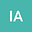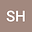Lucas and Fibonacci polynomials based approach for the study of one- and two-dimensional Burger and heat-type equations
••• Ihteram Ali,
• Sirajul Haq
Ihteram Ali
Ghulam Ishaq Khan Institute of Engineering Sciences and Technology

Corresponding Author:[email protected]

Author ProfileSirajul Haq
Ghulam Ishaq Khan Institute of Engineering Sciences and Technology
Author Profile## Abstract

In this work a numerical technique, combination of Lucas and Fibonacci polynomials, is proposed for the solution of one- and two-dimensional nonlinear heat type equations. In first round, for discretization, finite difference has been used for time and Crank Nicolson scheme for spatial part. In second round, the unknown functions have been approximated by Lucas polynomial while their derivatives by Fibonacci polynomials. With the help of these approximations, the nonlinear partial differential equation transforms to a system of algebraic equations which can be solved easily. Convergence of the method has been investigated numerically. Performance of the method has been studied by taking one- and two-dimensional heat and burger equations. Efficiency of the technique has been investigated in terms of root mean square (RMS), L2 and L_infnty norms. The obtained results are then compared with those available in the literature.